# Percentage of Bricks and Mortar in a Masonry Wall

By Jahanzaib
16-3-2015

### Case 1: When thickness of the wall is 4.5” and that of mortar is 12mm, having the following plan.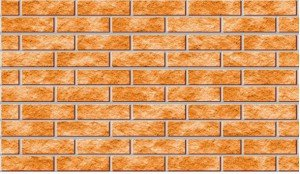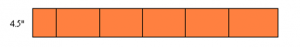In this case
Height of the wall along with the mortar = (10 x 3) + [(9 x 12)/25.4] = 34.252″
In the above equation
10 = No of Brick Layers
9 = No of layers of Mortar of 12mm each
3 = Brick Height in inches
Width=   4.5”
Length of the wall along with the mortar= (5.5 x 9) + [(5×12)/25.4] Volume of the wall along with the mortar= L×W×H
Volume of the wall along with the mortar= 7993.72 cubic inch
Volume of the wall along with the mortar= 4.626 cft
Now Number of Bricks= 55
Volume of One Brick= (9/128) cft
Total volume of bricks used= 55 x (9/128) = 3.867 cft
%age of bricks used = (3.867 / 4.626)*100 = 83.6 = 84%
%age of mortar used= 16.4%

### Case 2:When thickness of the wall is 9” and that of mortar is 12mm, having the following plan.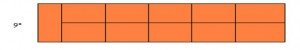In this case
Height of the wall along with the mortar = (10 x 3 ) + [( 9 x 12 )/25.4] = 34.252″
Width of the wall along with the mortar = (9+12/25.4)” = 9.472″
Length of the wall along with the mortar= (5.5 x 9) + (5 x 12/25.4) = 51.862″
Volume of the wall along with the mortar= L×W×H
Volume of the wall along with the mortar=16826.678 cubic inch
Volume of the wall along with the mortar= 9.7376 cft
Now Number of Bricks= 110
Volume of One Brick= 9/128 cft
Total volume of bricks used= 110 x (9/128) = 7.7344 cft
So
%age of bricks used = (7.7344 / 9.7376) x 100 = 79.43% = 80%
%age of mortar used= 20.57% = 21%

### Case 3: When thickness of the wall is 4.5” and that of mortar is 10mm, having the following plan.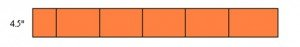In this case
Height of the wall along with the mortar = (10 x 3) + (9 x 10/25.4) = 33.543″
width = 4.5″
Length of the wall along with the mortar= (5.5 x 9) + (5 x 10/25.4) = 51.468″
Volume of the wall along with the mortar= L×W×H
Volume of the wall along with the mortar= 4.4959 cft
Now Number of Bricks= 55
Volume of One Brick= 9/128 cft
Total volume of bricks used= 55 x 9/128 = 3.8672 cft
%age of bricks used = (3.8672 / 4.4959) x 100 = 86%
%age of mortar used = 14%

### Case 4:When thickness of the wall is 9” and that of mortar is 10mm, having the following plan.###In this case
Height of the wall along with the mortar = (10 x 3) + (9 x 10/25.4) = 33.543″
width = (9 + 10/25.4)” 9.3937″
Length of the wall along with the mortar= (5.5 x 9) + (5 x 10/25.4) = 51.468″
Volume of the wall along with the mortar= L×W×H
Volume of the wall along with the mortar= 9.38513 cft
Now Number of Bricks= 110
Volume of One Brick= 9/128 cft
Total volume of bricks used= 110 x 9/128 = 7.7344 cft
%age of bricks used = (7.7344 / 9.38513) x 100 = 82.4% = 82%
%age of mortar used = 17.6% = 18%

### Conclusion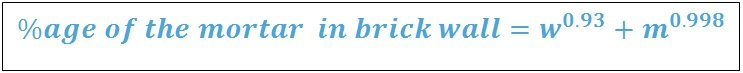Where in it
W= wall thickness in inches
m= thickness of the mortar in mm
The answer will be the %age of mortar, and for better results round it off.
Its Verification is given below after the example from observation.

EXAMPLE:
If thickness of the wall is 4.5” and that of mortar is 12mm, then what will the percentage  of mortar used in the wall?

Solution: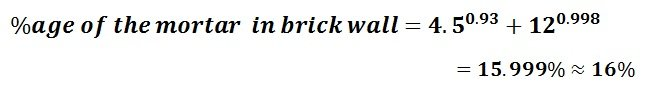Want to write articles or share your thoughts/experience or any other interesting thing ? then contact us at  civilengineerspk@gmail.com

## 13 Replies to “Percentage of Bricks and Mortar in a Masonry Wall”

1.shehbaz on said:

is this formula also useful to calculate the percentages of mix design mortar..e.g when in cement sand there is also present a third quantity to increase the strength of the mortar..

•UBAIDAHMAD on said:

That is Crush the Third quantity. You will have to do Proper Rate Analysis to find them

2.alwin on said:

i learn so much as your website thank you

3.Iqbal on said:

•UBAIDAHMAD on said:

welcome

4.Azam on said:

I usually see and even read in quantity book even though not satisfied that in 100cft brickwork it required 1350 number of bricks and 30cft dry mortar is it correct plz guide

5.M Haris on said:

the bricks calculate in wall which formula to use plz tell me.

•CIVILENGINEERSPK on said:

Its written.

6.habib on said:

please explain this portion of case 1..
Now Number of Bricks= 55
Volume of One Brick= (9/128) cft
Total volume of bricks used= 55 x (9/128) = 3.867 cft

•Muhammad Amir on said:

Volume of One brick = 9/128
Brief
Volume of One Brick = 9″ x 4.5″ x 3″ (L X B X H)

7.kdugglus91 on said:

Thanks for sharing such a great knowledge with us, your post is great and your information give best result.

•CIVILENGINEERSPK on said:

welcome